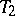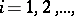# Compactum

(diff) ← Older revision | Latest revision (diff) | Newer revision → (diff)

A metrizable compact space. Examples of compacta are: a segment, a circle, an-dimensional cube, ball or sphere, the Cantor set, the Hilbert cube; an-dimensional Euclidean space is not a compactum, but a subset of it is a compactum if and only if it is closed and bounded. A closed subset of a compactum is a compactum and every compactum is homeomorphic to a closed subset of the Hilbert cube (Urysohn's theorem). In order that there exist a homeomorphism of a compactum into a Euclidean space, it is necessary and sufficient that the compactum be finite-dimensional (the Pontryagin–Nöbeling theorem). A continuous image of a compactum that is a-space is a compactum, and every compactum is the continuous image of the Cantor set (Aleksandrov's theorem). The product of a finite or countable set of compacta is a compactum. Every compactum is separable; among the Hausdorff compact spaces, the compacta are characterized by the property that they possess a finite or countable basis. A compactum is also characterized by the fact that it is totally bounded with respect to any metric compatible with its topology (Hausdorff's theorem).

The compacta form one of the most important classes of topological spaces. The property that a metrizable spacebe a compactum is equivalent to each of the following properties.

1) From any countable open covering of the spaceone can select a finite subcovering (an analogue of the Heine–Borel–Lebesgue covering theorem on covering a line segment by intervals; cf. also Borel–Lebesgue covering theorem).

2) Any countable system of non-empty closed subsetsofsuch that,has a non-empty intersection (a generalization of Cantor's principle of nested intervals).

3) Any sequence of points inhas a convergent subsequence in(a generalization of the Bolzano–Weierstrass theorem).

4) Any infinite subset ofhas at least one limit point in(a generalization of the Bolzano–Weierstrass theorem).

5) Any continuous function onis bounded (a generalization of Weierstrass' theorem).

6) Any continuous function onattains its maximum (minimum) value at some point (a generalization of Weierstrass' theorem).

7)is totally bounded and complete with respect to any metric compatible with its topology.

Any continuous function on a compactumis uniformly continuous with respect to any metric compatible with the topology of(a generalization of the Heine–Cantor theorem).

How to Cite This Entry:
Compactum. Encyclopedia of Mathematics. URL: http://encyclopediaofmath.org/index.php?title=Compactum&oldid=17404
This article was adapted from an original article by B.A. Pasynkov (originator), which appeared in Encyclopedia of Mathematics - ISBN 1402006098. See original article